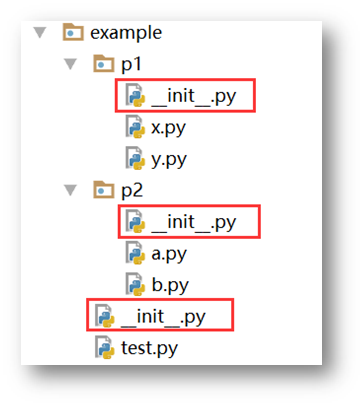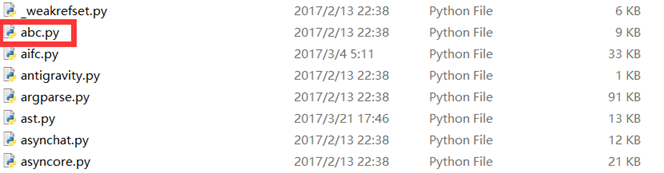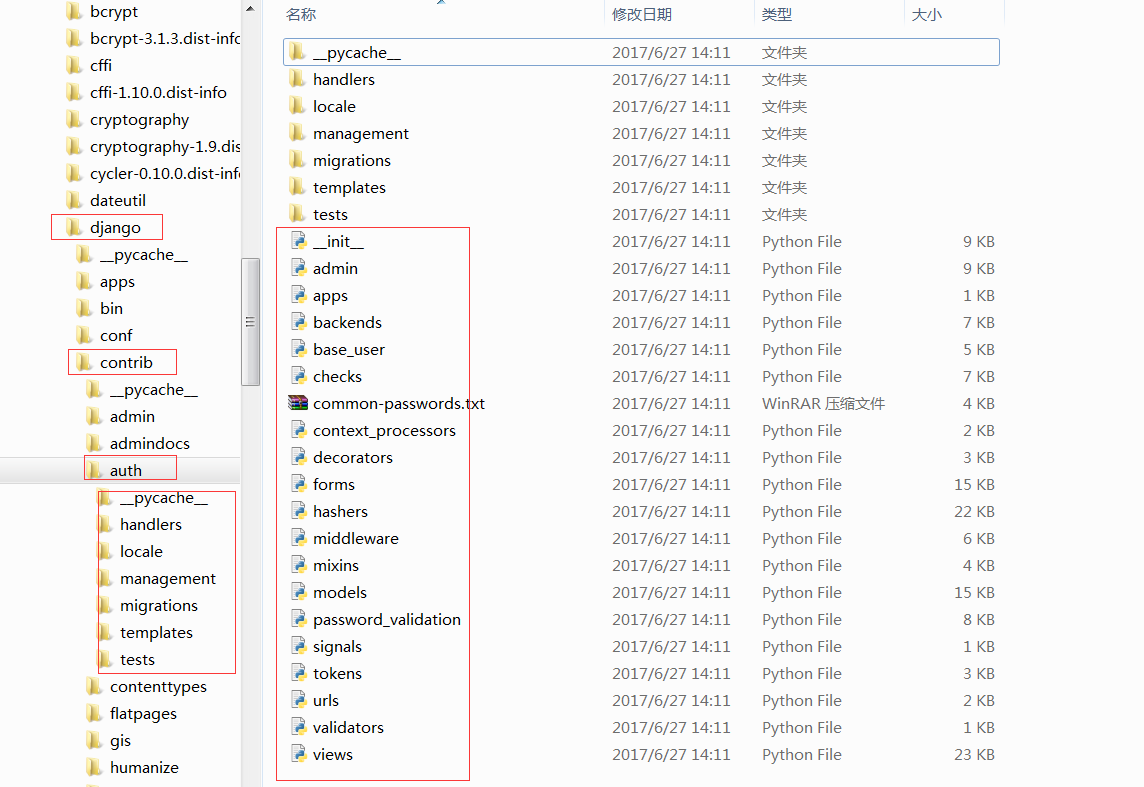# 模块与包

• 首先，提高了代码的可维护性。

• 其次，编写代码不必从零开始。当一个模块编写完毕，就可以被其他的模块引用。不要重复造轮子，我们简简单单地使用已经有的模块就好了。

• 使用模块还可以避免类名、函数名和变量名发生冲突。相同名字的类、函数和变量完全可以分别存在不同的模块中。但是也要注意尽量不要与内置函数名（类名）冲突。• `import xx.xx`
• `from xx.xx import xx`
• `from xx.xx import xx as rename`
• `from xx.xx import *`

### 1. `import xx.xx`

```# Module_a.py

def func():
print("this is module A!")
```

`Main.py`中导入`Module_a`

```# Main.py

import module_a

module_a.func()  # 调用方法
```

### 2. `From xx.xx import xx.xx`

`Main.py`中导入`Module_a`

```# Main.py

from module_a import func

module_a.func()   # 错误的调用方式

func()  # 这时需要直接调用func
```

### 3. `from xx.xx import xx as rename`

```# Main.py

from module_a import func as f

def func(): ## main模块内部已经有了func函数
print("this is main module!")

func()
f()
```

### 4. `from xx.xx import *`

```# Main.py

from module_a import *

def func():
print("this is main module!")

func()  # 从module导入的func被main的func覆盖了
```

### 模块搜索路径

Python根据`sys.path`的设置，按顺序搜索模块。

```>>> import sys
>>> sys.path
['', 'C:\\Python36\\Lib\\idlelib', 'C:\\Python36\\python36.zip', 'C:\\Python36\\DLLs', 'C:\\Python36\\lib', 'C:\\Python36', 'C:\\Python36\\lib\\site-packages']
```

```import sys
import os

new_path = os.path.abspath('../')
sys.path.append(new_path)
```

1. 当前执行脚本所在目录
2. Python的安装目录
3. Python安装目录里的site-packages目录

### 实例讲解

• 在Pycharm里建个py文件`abc.py`，写入代码：
```def my_abs():
print("my_abs!")

if __name__ == '__main__':
my_abs()
```
• 同级目录下再建个`main.py`，代码如下：
```from abc import my_abs
my_abs()
```
• 运行main.py，出错了，错误信息如下:
```Fatal Python error: Py_Initialize: can't initialize sys standard streams
Traceback (most recent call last):
File "C:\Python36\lib\io.py", line 72, in <module>
AttributeError: module 'abc' has no attribute 'ABCMeta'
```

```D:\>python abc.py
my_abs!

D:\>python main.py
Traceback (most recent call last):
File "main.py", line 2, in <module>
from abc import my_abs
ImportError: cannot import name 'my_abs'

D:\>python
Python 3.6.1 (v3.6.1:69c0db5, Mar 21 2017, 18:41:36) [MSC v.1900 64 bit (AMD64)] on win32
>>> from abc import my_abs
Traceback (most recent call last):
File "<stdin>", line 1, in <module>
ImportError: cannot import name 'my_abs'
```

1. Python有个内置模块`abc.py`，是不是这个原因呢？在Python的lib文件夹中可以找到如下图的内置标准模块`abc.py`文件。

2. 可是我们是在同级目录下导入`abc`的，按照前面的模块查询顺序，不是先找本地目录吗？

3. 按照2的分析，自定义的`abc.py`文件会短路掉Python内置的`abc`模块，不应该有问题。(虽然这是不好的做法，但从程序角度上没问题。)

4. 通过查看源码发现真实的原因是print语句需要调用io模块，而io模块又要调用Python内置的abc模块，但此时的abc命名空间被自定义的模块覆盖了，因而发生错误。## 包（Package）：`__init__.py`可以是空文件，也可以有Python代码，`__init__.py`本身就是一个模块，但是要注意，它的模块名是它所在的包名而不是`__init__`

```# example\p1\x.py

def show():
print("this is module x")
```
```# example\p2\a.py

import example.p1.x

def show():
print("this is modula a")

example.p1.x.show()
show()
```

```this is module x
this is modula a
```

Python会进入文件系统，找到这个包里面所有的子模块，一个一个的把它们都导入进来。 但是这个方法有风险，有可能导入的模块和已有的模块冲突，或者并不需要导入所有的模块。为了解决这个问题，需要提供一个精确的模块索引。这个索引要放置在`__init__.py`中。

`__all__ = ["x"]`

#### 评论总数： 2